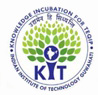## EventsTEQIP III National Short Term Course on

"Computer Aided Applied Numerical Analysis and Optimization Techniques"

(January 17 - 21, 2020)The course would provide knowledge in numerical and optimization techniques. The proposed course is not specific to any single discipline and can attract participants from various branches of science, engineering and management. Numerical problems such as solution of simultaneous linear equations, solution of non-linear equations, linear and non-linear regression, polynomial fitting, splines, ordinary differential equations, partial differential equations would be taught as part of the course. The participants would also be provided with hands on sessions which will enable them to quickly solve large scale problems using state-of-the-art-software. The optimization techniques will comprise mathematical programming (MP) as well as computational intelligence techniques (CIT) so as to solve optimization problems in the context of the availability of a well-defined mathematical model and also in the context of the model being a black box. In Mathematical Programming Techniques, the participants will learn to solve linear programming problems (LP), non-linear programming problems (NLP), mixed integer linear programming problems (MILP) and also mixed-integer non-linear programming problems (MINLPs). They will also be given exposure to state-of-the-art optimization tools in this domain through hands-on training sessions. In computational intelligence techniques, the course will cover the evolutionary as well as swarm optimization techniques. In particular, the focus will be on conventional techniques such as Genetic Algorithm (GA), Particle Swarm Optimization (PSO), Differential Evolution (DE) and Artificial Bee Colony (ABC). In addition, the participants would be introduced to recently proposed techniques such as Sanitized Teaching Learning Based Optimization (s-TLBO) and Yin-Yang Pair Optimization (YYPO). The candidates would also get knowledge on the performance evaluation of single objective optimization techniques. The course will also lay emphasis on the development of single objective optimization algorithms and will encourage participants to conceive a single objective optimization algorithm on their own. The course will also cover solution of multi-objective optimization problems. The course will also address various other aspects such as handling of constraints using penalty function approach and also focus on various types of special variables such as integer and semi-continuous variables which are widely encountered in real-life optimization problems. A unique feature of the course, which is not covered in most textbooks, is that it will lay emphasis on appropriate modelling of the problem with respect to mathematical programming and computational intelligence techniques. The participants will be taught on the judicious use of appropriate variables and constraints to enable the development of a suitable model for the two categories of optimization techniques. The course will employ two complex combinatorial optimization problems viz., production planning and job-shop scheduling to demonstrate the appropriate modelling for solving using mathematical programming as well as computational intelligence techniques. The participants will be introduced to state-of-the-art computational tools for numerical and optimziation techniques such as MATLAB, IBM ILOG CPLEX Optimization Studio and General Algebraic Modelling System.